# Huygens Principle

Huygen’s principle is one of the important principles when you are studying about waves and conducting their analysis. Huygen’s principle basically helps us to understand as well as describe the movements of waves. If you are familiar with the concept of waves, then you must have already learnt that their behaviour can sometimes be unreasonable.

Waves generally do not move in a straight line and they have got certain characteristics that allow them to move in all directions. In 1678, Huygens suggested that each point on a wave’s wavefront was a source of a spherical wave. The resultant wave is determined by adding all waves from the point of sources. Well, Huygen’s principle helps us to understand such factors and the reason behind the particular behaviour of the waves.

## Huygens Principle of Secondary Wavelets – Statement

Huygen’s principle also sometimes called as Huygens–Fresnel principle states that each point on a given wavefront is a source of secondary wavelets or secondary disturbances. Further, the disturbances originating from the secondary source spreads in all directions in the same way as originating from the primary source.

This principle further highlights things such as;

• Secondary sources start making their own wavelets, these waves are similar as of primary source.
• At any instant of time, common tangent on the wavelets in the forward direction gives the new wavefront.
• The sum of spherical wavelets forms the wavefront.

In essence, Huygen’s principle is a comprehensive method of analysis that can be used to understand the problems of wave propagation both in diffraction and reflection.

Also Read: Young’s Double Slit Experiment

### Examples of Huygen’s Principle

• If stone is thrown into the river it will create waves around that point.
• These waves look like circular rings and are called wavefront waves.
• Gradually, the wavefronts disperse in all directions.
• When the locus of all the waves in the same phase is joined, it is the same as a sphere and is known as the primary wavefront.
• Secondary wavefront is generated from each point on the primary wavefront.
• Moreover, the rising tangential line enveloping these secondary wavefronts can give rise to other secondary wavefronts.
• After some time all the wavefronts will slowly disappear.

## Outcomes of Huygen’s Principle

Some of the outcomes of the principle are;

• Huygens wave principle proved the concept of reflection of light.
• The principle also approved the concept of refraction of light.
• It proved the concept of interference of light and the concept of diffraction of light.

However, Huygen’s principle failed to prove the concept of polarization of light, emission of light, absorption of light and the photoelectric effect.

## Huygen’s Theory Important Considerations

Below are some of the important considerations of Huygen’s principle.

1. All the rays are always perpendicular to the wavefront.
2. Time taken by a wave from one wavefront to another wavefront is always constant. In the case of different medium, distance and velocity can change but time will always remain constant.
3. All the points on a wavefront act like a secondary source known as secondary wavelets.

### Wavefront

Locus of all the points which are in the same phase is known as a wavefront. There are different types of wavefront which we will discuss below.

Spherical Wavefront

In case of a point source radiating energy, the particle around the energy begins to oscillate, these waves travel in all directions and form a spherical wavefront.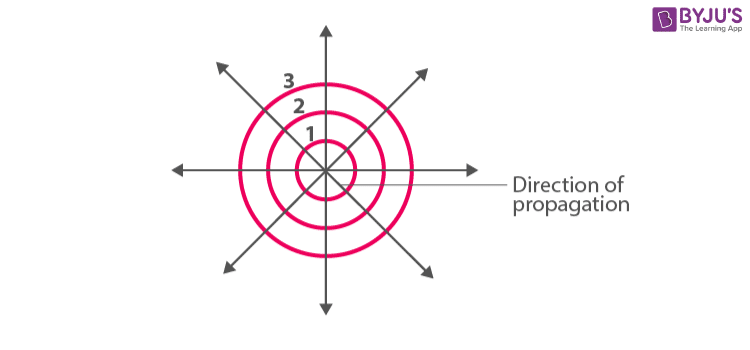Planer Wavefront

If the source is at infinity then the waves coming out from the source are parallel to each other (light rays coming from the sun are parallel to each other on earth). In such cases, there is a formation of planer wavefront.

We can convert planer wavefront and spherical wavefront in the following manner.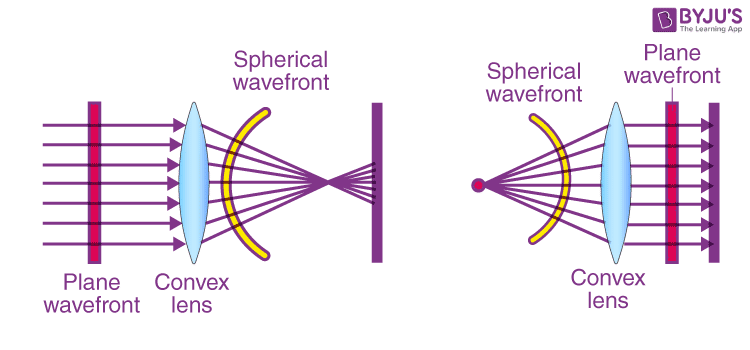Cylindrical Wavefront

Cylindrical wavefront is formed if the light source is linear. Here, all the points will be equidistant from the source and we will find them on the surface of a cylinder.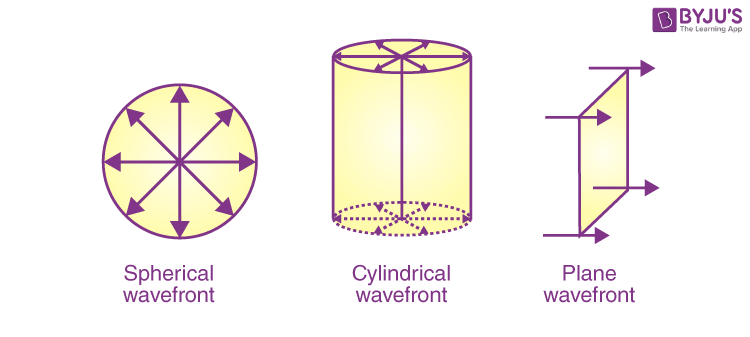The difference in the aptitude and intensity between three types of wavefronts

 Wavefront types Amplitude Intensity Spherical wavefront A ∝ 1/r I ∝ 1/r2 Planar wavefront A ∝ r0 I ∝ r0 Cylindrical wavefront A ∝ 1/√r I ∝ 1/r

## Proof of Reflection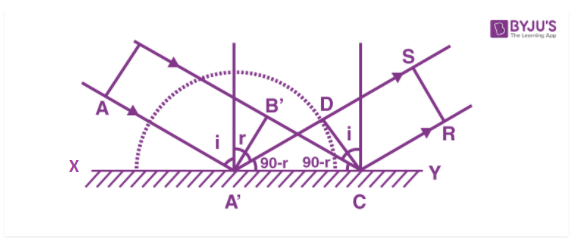If you look at the figure, AA’ is the wavefront incident on a reflecting surface XY having an angle of incidence i. Following Huygen’s principle, every point on AA’ will act as a source of secondary wavelets.

Time taken from A’ to D = time taken from B’ to C

A’D / v = B’C / v

A’D = B’C

A’C sin(i) = A’C sin(r)

Thus, i = r

Hence, the angle of incidence and angle of reflection are both equal. This is also stated by the first law of reflection. Additionally, as the incident wavefront AB, the normal and reflected wavefront are on the same plane, we can also verify the second law of reflection.

## Proof of Refraction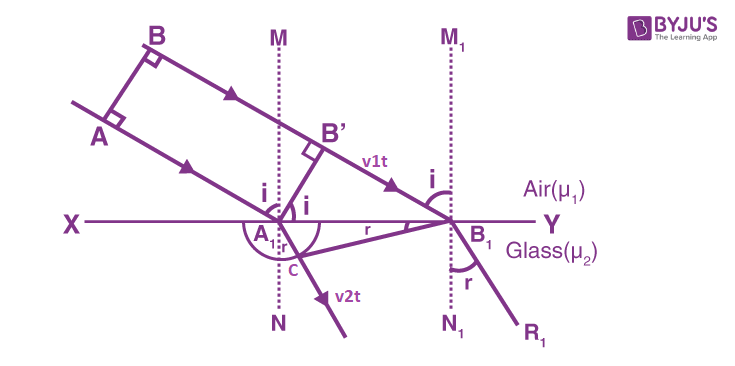Let the angle of incidence be i and the refraction is r.

From the ΔA1B’B1, we get

Sin∠B’A1B1 = Sin i = B’B/AB1

From the ΔA1CB1, we get

Sin∠A1B1C = Sin r = A1C/AB1

Sin i/Sin r = B’B/A1C

= v1t/v2t

Sin i/Sin r = v1/v= 1μ2(constant)

This proves Snell’s law of refraction. The constant 1μis called the refractive index of the second medium w.r.t the first medium.

## Applications Of Huygen’s Theory

By using Huygen’s theory after time t, we can determine the new location of the wavefront. Mathematically we can also calculate the new position of wavefront after time t.

1. Let the initial time ti = 0.
2. Distance travelled by the individual wave is equal to the sphere radius.